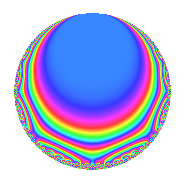# Properties

 Label 55.2.e.bLevel 55 Weight 2 Character orbit 55.e Analytic conductor 0.439 Analytic rank 0 Dimension 4 CM disc. -11 Inner twists 4

# Related objects

## Newspace parameters

 Level: $$N$$ = $$55 = 5 \cdot 11$$ Weight: $$k$$ = $$2$$ Character orbit: $$[\chi]$$ = 55.e (of order $$4$$ and degree $$2$$)

## Newform invariants

 Self dual: No Analytic conductor: $$0.439177211117$$ Analytic rank: $$0$$ Dimension: $$4$$ Relative dimension: $$2$$ over $$\Q(i)$$ Coefficient field: $$\Q(i, \sqrt{11})$$ Coefficient ring: $$\Z[a_1, \ldots, a_{5}]$$ Coefficient ring index: $$1$$ Sato-Tate group: $\mathrm{U}(1)[D_{4}]$

## $q$-expansion

Coefficients of the $$q$$-expansion are expressed in terms of a basis $$1,\beta_1,\beta_2,\beta_3$$ for the coefficient ring described below. We also show the integral $$q$$-expansion of the trace form.

 $$f(q)$$ $$=$$ $$q + ( -1 + \beta_{1} - \beta_{3} ) q^{3} + 2 \beta_{2} q^{4} + ( -\beta_{1} - \beta_{2} ) q^{5} + ( 1 - 3 \beta_{2} + 2 \beta_{3} ) q^{9} +O(q^{10})$$ $$q + ( -1 + \beta_{1} - \beta_{3} ) q^{3} + 2 \beta_{2} q^{4} + ( -\beta_{1} - \beta_{2} ) q^{5} + ( 1 - 3 \beta_{2} + 2 \beta_{3} ) q^{9} + ( -2 \beta_{1} + \beta_{2} ) q^{11} + ( 2 \beta_{1} - 2 \beta_{2} + 2 \beta_{3} ) q^{12} + ( -3 - \beta_{1} + 4 \beta_{2} - 2 \beta_{3} ) q^{15} -4 q^{16} + ( 2 - 2 \beta_{3} ) q^{20} + ( 4 + \beta_{1} - 5 \beta_{2} - \beta_{3} ) q^{23} + ( 2 + 3 \beta_{3} ) q^{25} + ( 5 - \beta_{1} + 6 \beta_{2} - \beta_{3} ) q^{27} + ( 6 \beta_{1} - 3 \beta_{2} ) q^{31} + ( -6 + \beta_{1} + 5 \beta_{2} - \beta_{3} ) q^{33} + ( 6 - 4 \beta_{1} + 2 \beta_{2} ) q^{36} + ( -5 - 3 \beta_{1} - 2 \beta_{2} - 3 \beta_{3} ) q^{37} + ( -2 - 4 \beta_{3} ) q^{44} + ( -3 + 3 \beta_{1} - 7 \beta_{2} + 3 \beta_{3} ) q^{45} + ( -5 + 2 \beta_{1} - 7 \beta_{2} + 2 \beta_{3} ) q^{47} + ( 4 - 4 \beta_{1} + 4 \beta_{3} ) q^{48} + 7 \beta_{2} q^{49} + ( -1 - 4 \beta_{1} + 5 \beta_{2} + 4 \beta_{3} ) q^{53} + ( 7 + 3 \beta_{3} ) q^{55} + ( 1 + 2 \beta_{3} ) q^{59} + ( -8 + 4 \beta_{1} - 6 \beta_{2} - 2 \beta_{3} ) q^{60} -8 \beta_{2} q^{64} + ( 5 - 3 \beta_{1} + 8 \beta_{2} - 3 \beta_{3} ) q^{67} + ( -4 - \beta_{2} - 8 \beta_{3} ) q^{69} -3 q^{71} + ( 7 - \beta_{1} + 9 \beta_{2} - 2 \beta_{3} ) q^{75} + ( 4 \beta_{1} + 4 \beta_{2} ) q^{80} + ( -2 + 6 \beta_{1} - 3 \beta_{2} ) q^{81} + 9 \beta_{2} q^{89} + ( 10 + 2 \beta_{1} + 8 \beta_{2} + 2 \beta_{3} ) q^{92} + ( 18 - 3 \beta_{1} - 15 \beta_{2} + 3 \beta_{3} ) q^{93} + ( -10 - 3 \beta_{1} - 7 \beta_{2} - 3 \beta_{3} ) q^{97} + ( 3 - 11 \beta_{2} + 6 \beta_{3} ) q^{99} +O(q^{100})$$ $$\operatorname{Tr}(f)(q)$$ $$=$$ $$4q - 2q^{3} + O(q^{10})$$ $$4q - 2q^{3} - 4q^{12} - 8q^{15} - 16q^{16} + 12q^{20} + 18q^{23} + 2q^{25} + 22q^{27} - 22q^{33} + 24q^{36} - 14q^{37} - 18q^{45} - 24q^{47} + 8q^{48} - 12q^{53} + 22q^{55} - 28q^{60} + 26q^{67} - 12q^{71} + 32q^{75} - 8q^{81} + 36q^{92} + 66q^{93} - 34q^{97} + O(q^{100})$$

Basis of coefficient ring in terms of a root $$\nu$$ of $$x^{4} - 5 x^{2} + 9$$:

 $$\beta_{0}$$ $$=$$ $$1$$ $$\beta_{1}$$ $$=$$ $$\nu$$ $$\beta_{2}$$ $$=$$ $$($$$$\nu^{3} - 2 \nu$$$$)/3$$ $$\beta_{3}$$ $$=$$ $$\nu^{2} - 3$$
 $$1$$ $$=$$ $$\beta_0$$ $$\nu$$ $$=$$ $$\beta_{1}$$ $$\nu^{2}$$ $$=$$ $$\beta_{3} + 3$$ $$\nu^{3}$$ $$=$$ $$3 \beta_{2} + 2 \beta_{1}$$

## Character Values

We give the values of $$\chi$$ on generators for $$\left(\mathbb{Z}/55\mathbb{Z}\right)^\times$$.

 $$n$$ $$12$$ $$46$$ $$\chi(n)$$ $$\beta_{2}$$ $$-1$$

## Embeddings

For each embedding $$\iota_m$$ of the coefficient field, the values $$\iota_m(a_n)$$ are shown below.

For more information on an embedded modular form you can click on its label.

Label $$\iota_m(\nu)$$ $$a_{2}$$ $$a_{3}$$ $$a_{4}$$ $$a_{5}$$ $$a_{6}$$ $$a_{7}$$ $$a_{8}$$ $$a_{9}$$ $$a_{10}$$
32.1
 −1.65831 + 0.500000i 1.65831 + 0.500000i −1.65831 − 0.500000i 1.65831 − 0.500000i
0 −2.15831 + 2.15831i 2.00000i 1.65831 1.50000i 0 0 0 6.31662i 0
32.2 0 1.15831 1.15831i 2.00000i −1.65831 1.50000i 0 0 0 0.316625i 0
43.1 0 −2.15831 2.15831i 2.00000i 1.65831 + 1.50000i 0 0 0 6.31662i 0
43.2 0 1.15831 + 1.15831i 2.00000i −1.65831 + 1.50000i 0 0 0 0.316625i 0
 $$n$$: e.g. 2-40 or 990-1000 Significant digits: Format: Complex embeddings Normalized embeddings Satake parameters Satake angles

## Inner twists

Char. orbit Parity Mult. Self Twist Proved
1.a Even 1 trivial yes
11.b Odd 1 CM by $$\Q(\sqrt{-11})$$ yes
5.c Odd 1 yes
55.e Even 1 yes

## Hecke kernels

This newform can be constructed as the kernel of the linear operator $$T_{2}$$ acting on $$S_{2}^{\mathrm{new}}(55, [\chi])$$.